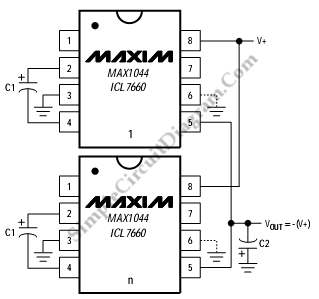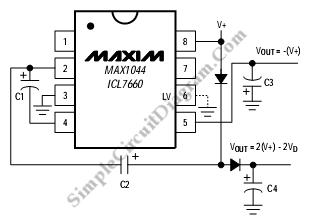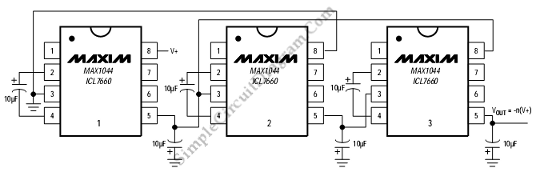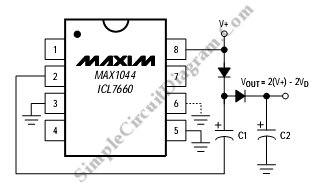## Paralleling MAX1044 DevicesThis is a circuit of Paralleling devices using MAX1044. This circuit can be used to increase current capability and reduce output resistance. In this circuit capacitor C1 is used as pump and C2 used as reservoir that serves all devices. Here is the circuit : The output resistance is defined by following equation : Rout=Rout of MAX1044 or ICL7660s/n [Source: […]

Read more

## Combined Positive Multiplication and Negative Voltage ConversionThis is a Combined Positive Multiplication and Negative Voltage Conversion circuit, a popular application for MAX1044/ICL7660. Here is the circuit: This circuit can provides both negative and positive voltage. TO provide negative voltage, this circuit uses C3 and C1 which perform the reservoir and bucket. To generate the doubled positive voltage, the C4 and C2 are used as the reservoir […]

Read more

## Cascading MAX1044/ICL7660 Voltage Doubler DevicesThis is a circuit of  Cascading Devices using MAX1044/ICL7660. This circuit can provide Larger negative multiples of the supply voltage. The resistance of first that multiplied by the quiescent current of the second, etc will reduce the output voltage. The devices that can be cascaded is not only two devices but also three or more devices. However the output impedance […]

Read more

## Positive Voltage DoublerThis is a simple circuit of Positive Voltage Doubler. This circuit uses the MAX1044/ICL7660. The schottky diode was chosen to reduces the voltage drop. This circuit also uses MAX660 for voltage doubling or higher output currents. Here is the circuit: [Source: Maxim Application Note]

Read more